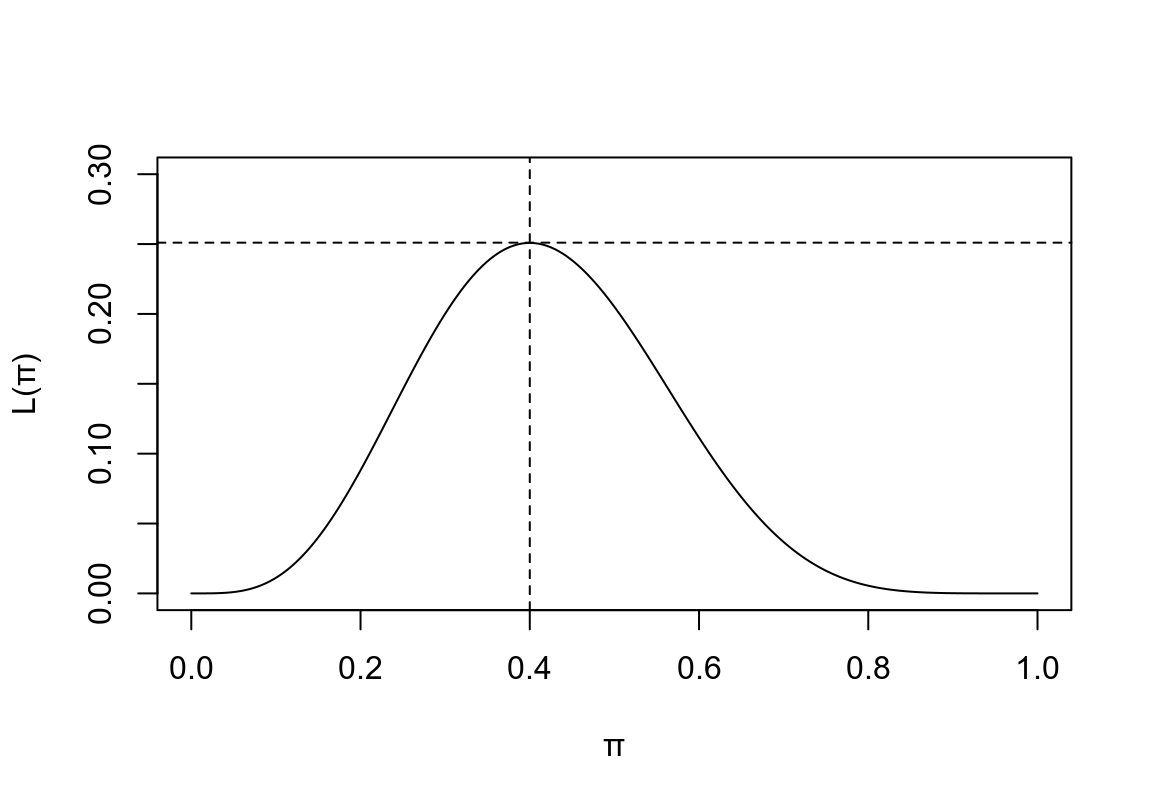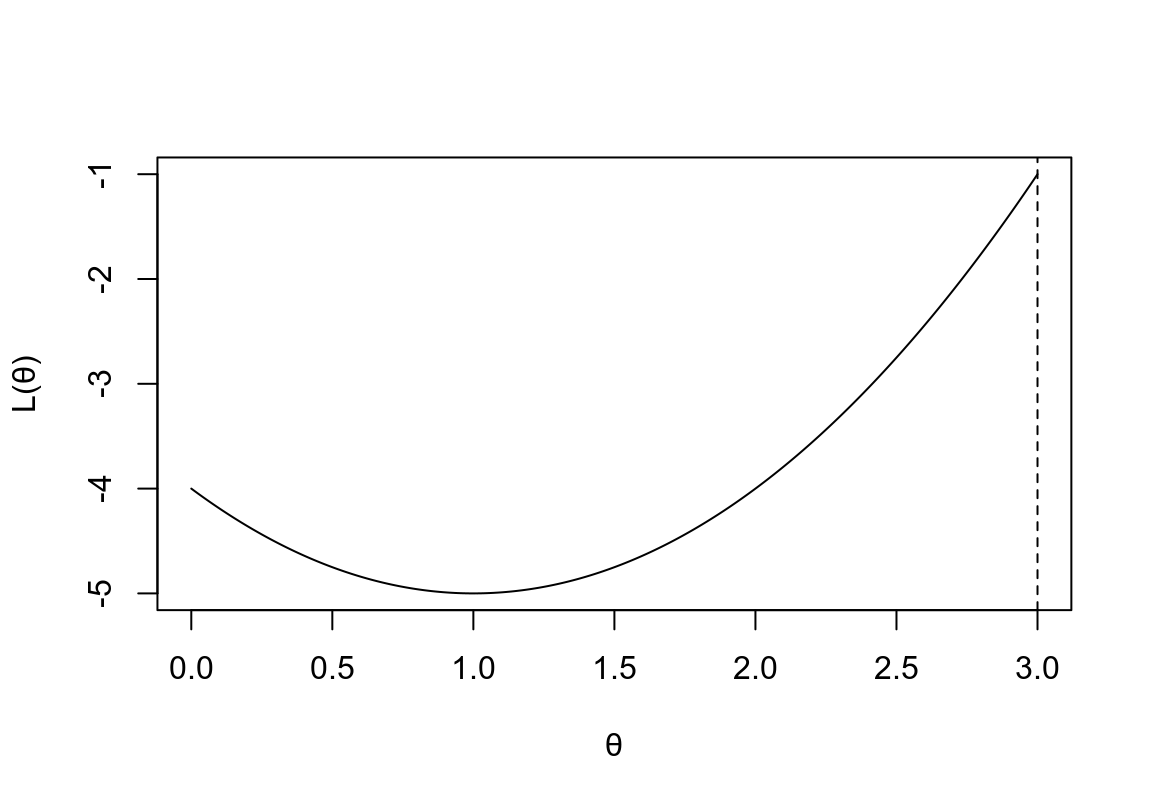# 第 12 章 似然 Likelihood

## 12.1 概率 vs. 推斷 Probability vs. Inference

$\binom{10}{4}\times(0.5^4)\times(0.5^{10-4}) = 0.205$

dbinom(4, 10, 0.5)
##  0.2051
# or you can calculate by hand:
factorial(10)*(0.5^10)/(factorial(4)*(factorial(6)))
##  0.2051

## 12.2 似然和極大似然估計 Likelihood and maximum likelihood estimators

$Prob(X=4|\pi)=\binom{10}{4}\pi^4(1-\pi)^{10-4}$

Table 12.1: The probability of observing $$X=4$$
$$\pi$$ 事件 $$X=4$$ 發生的概率
0.0 0.000
0.2 0.088
0.4 0.251
0.5 0.205
0.6 0.111
0.8 0.006
1.0 0.000

• 觀察到實驗數據(10人中4個患病) ；
• 假定這數據服從二項分佈的概率模型，計算不同($$\pi$$ 的取值不同的) 情況下，該事件按照假定模型發生的概率；
• 通過比較，我們選擇了能夠讓觀察事件發生概率最高的參數取值 ($$\pi=0.4$$)。

$L(\pi|X=4)=\binom{10}{4}\pi^4(1-\pi)^{10-4}$

x <- seq(0,1,by=0.001)
y <- (factorial(10)/(factorial(4)*(factorial(6))))*(x^4)*((1-x)^6)
plot(x, y, type = "l", ylim = c(0,0.3), ylab = "L(\U03C0)", xlab = "\U03C0")
#title("Figure 1. Binomial Likelihood")
abline(h=0.251, lty=2)
abline(v=0.4, lty=2)## 12.3 似然方程的一般化定義

$$L(\theta|\underline{x})=P(\underline{x}|\theta)$$

1. $$P(\underline{x}|\theta)$$ 可以是概率(離散分佈) 方程，也可以是概率密度(連續型變量) 方程。對於此方程，$$\theta$$ 是給定的，然後再計算某些事件發生的概率。
2. $$L(\theta|\underline{x})$$ 是一個關於參數 $$\theta$$ 的方程，此時，$$\underline{x}$$ 是固定不變的(觀察值) 。我們希望通過這個方程求出能夠使觀察到的事件發生概率最大的參數值。
3. 似然方程不是一個概率密度方程。

Exercise 12.3
$$x$$ $$f(x|1)$$ $$f(x|2)$$ $$f(x|3)$$
0 1/3 1/4 0
1 1/3 1/4 0
2 0 1/4 1/6
3 1/6 1/4 1/2
4 1/6 0 1/3
$$x$$ $$f(x|1)$$ $$f(x|2)$$ $$f(x|3)$$ $$\theta$$
0 1/3 1/4 0 1
1 1/3 1/4 0 1
2 0 1/4 1/6 2
3 1/6 1/4 1/2 3
4 1/6 0 1/3 3

## 12.4 對數似然方程 log-likelihood

$\frac{d\ell}{d\theta}=\ell^\prime(\theta)=0\\ AND\\ \frac{d^2\ell}{d\theta^2}<0$

x <- seq(0,3,by=0.001)
y <- (x-1)^2-5
plot(x, y, type = "l", ylim = c(-5,0-1), ylab = "L(\U03B8)", xlab = "\U03B8")
#title("Figure 2. Likelihood function with \n a limited domain")
abline(v=3, lty=2)$\frac{dL}{d\theta}=0, \frac{d^2L}{d\theta^2}<0 \Rightarrow \theta=\hat{\theta}$

$$\ell=\text{log}L$$ 那麼 $$\frac{d\ell}{dL}=\ell^\prime=\frac{1}{L}$$

$\frac{d\ell}{d\theta}=\frac{d\ell}{dL}\cdot\frac{dL}{d\theta}=\frac{1}{L}\cdot\frac{dL}{d\theta}$

$$\ell(\theta|data)$$ 取最大值時：

$\frac{d\ell}{d\theta}=0\Leftrightarrow\frac{1}{L}\cdot\frac{dL}{d\theta}=0\\ \because \frac{1}{L}\neq0 \\ \therefore \frac{dL}{d\theta}=0\\ \Leftrightarrow \theta=\hat{\theta}$

\begin{aligned} \frac{d^2\ell}{d\theta^2} &= \frac{d}{d\theta}(\frac{d\ell}{dL}\cdot\frac{dL}{d\theta})\\ &= \frac{d\ell}{dL}\cdot\frac{d^2L}{d\theta^2} + \frac{dL}{d\theta}\cdot\frac{d}{d\theta}(\frac{d\ell}{dL}) \end{aligned}

$$\theta=\hat{\theta}$$ 時，$$\frac{dL}{d\theta}=0$$$$\frac{d^2L}{d\theta^2}<0 \Rightarrow \frac{d^2\ell}{d\theta^2}<0$$

## 12.5 極大似然估計 (maximum likelihood estimator, MLE) 的性質：

1. 漸進無偏 Asymptotically unbiased:
$$n\rightarrow \infty \Rightarrow E(\hat{\Theta}) \rightarrow \theta$$
2. 漸進最高效能 Asymptotically efficient:
$$n\rightarrow \infty \Rightarrow Var(\hat{\Theta})$$ 是所有參數中方差最小的估計
3. 漸進正態分佈 Asymptotically normal:
$$n\rightarrow \infty \Rightarrow \hat{\Theta} \sim N(\theta, Var(\hat{\Theta}))$$
4. 變形後依然保持不變 Transformation invariant:
$$\hat{\Theta}$$$$\theta$$ 的MLE時 $$\Rightarrow g(\hat{\Theta})$$$$g(\theta)$$ 的 MLE
5. 信息足夠充分 Sufficient：
$$\hat{\Theta}$$ 包含了觀察數據中所有的能夠用於估計參數的信息
6. 始終不變 consistent:
$$n\rightarrow\infty\Rightarrow\hat{\Theta}\rightarrow\theta$$ 或者可以寫成：$$\varepsilon>0, lim_{n\rightarrow\infty}P(|\hat{\Theta}-\theta|>\varepsilon)=0$$

## 12.6 率的似然估計 Likelihood for a rate

$D\sim Poi(\mu)$

$Prob(D=d)=e^{-\mu}\frac{\mu^d}{d!}=e^{-\lambda p}\frac{\lambda^dp^d}{d!}$

$L(\lambda|observed \;data)=e^{-\lambda p}\frac{\lambda^dp^d}{d!}$

\begin{aligned} \ell(\lambda|observed\;data) &= \text{log}(e^{-\lambda p}\frac{\lambda^dp^d}{d!}) \\ &= -\lambda p+d\:\text{log}(\lambda)+d\:\text{log}(p)-\text{log}(d!) \\ \end{aligned}

$$\ell^\prime(\lambda|data)=0$$:

\begin{aligned} \ell^\prime(\lambda|data) &= -p+\frac{d}{\lambda}=0\\ \Rightarrow \hat{\lambda} &= \frac{d}{p} \\ \end{aligned}

## 12.7 有 $$n$$ 個獨立觀察時的似然方程和對數似然方程

$L(\theta|x_1,\cdots,x_n)=f(x_1,\cdots,x_n|\theta)=\prod_{i=1}^nf(x_i|\theta)\\ \Rightarrow \ell(\theta|x_1,\cdots,x_n)=\sum_{i=1}^n\text{log}(f(x_i|\theta))$